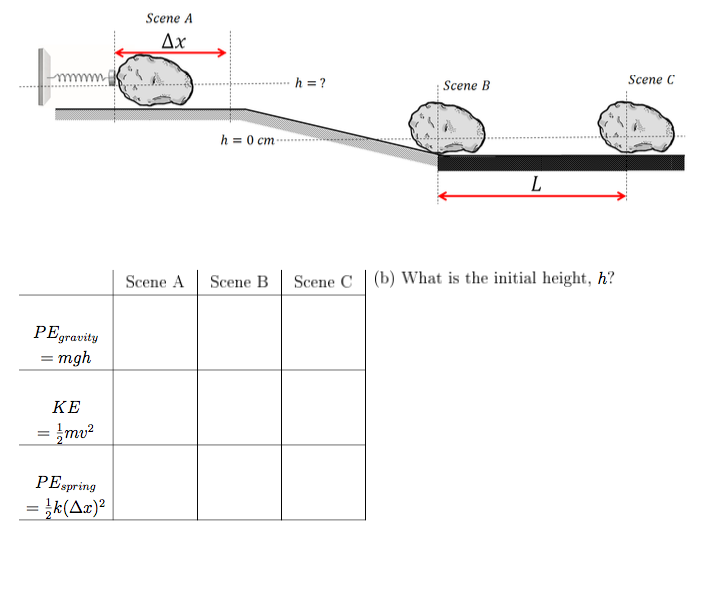# Scene A Ax Scene C h =? Scene B h 0 cm (b) What is the initial height, h? Scene B Scene A Scene C PEgravity mgh KE = }mu2 PEspring

Question

A 2.0 kg rock is pressed up a distance ∆x = 20 cm against a spring with spring constant 400 N/m and then released, causing it to slide across a frictionless horizontal surface and then to slide down a frictonless incline. At the bottom of the incline is a horizontal surface that has a coe cent of kinetic friction μk = 0.80 with the rock, which causes the rock to come to rest in L = 1.5 m. (a) Fill out the Energy Chart below to calculate the Potential and Kinetic Energy at each time point. Use g = 10 m/s2 for easier calcuations. Assume Energy is Conserved UNTIL the rock reaches the rough surface.help_outlineImage TranscriptioncloseScene A Ax Scene C h =? Scene B h 0 cm (b) What is the initial height, h? Scene B Scene A Scene C PEgravity mgh KE = }mu2 PEspring fullscreen